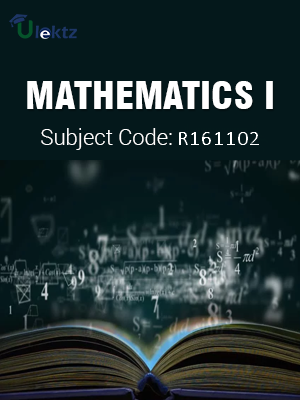•My WalletMy Order
•My Profile
•My Connections
•My Books
•My Videos
•My Tests
•My Calender
•My Messages
•My Shopping Cart
•My Orders
•Account Settings
•Help

# Book Details# MATHEMATICS I

 Course Code : R161102 Author : uLektz University : JNTU Kakinada Regulation : 2016 Categories : General Engineering Format :ePUB3 (DRM Protected) Type : eBook

FREE

Description :MATHEMATICS I of R161102 covers the latest syllabus prescribed by JNTU Kakinada for regulation 2016. Author: uLektz, Published by uLektz Learning Solutions Private Limited.

Note : No printed book. Only ebook. Access eBook using uLektz apps for Android, iOS and Windows Desktop PC.

##### Topics
###### Unit I: Differential equations of first order and first degree

1.1 Linear equation

1.2 Bernoulli equation

1.3 Exact-Reducible to exact.

1.4 Applications : Newton’s Law of cooling-Law of natural growth and decay orthogonal trajectories.

1.5 Electrical circuits- Chemical reactions

###### Unit II: Linear differential equations of higher order

2.1 Non-homogeneous equations of higher order with constant coefficients with RHS term of the type eax, sin ax, cos ax, polynomials in x, eax V(x), xV(x)

2.2 Method of Variation of parameters

2.3 Applications: LCR circuit, Simple Harmonic motion

###### Unit III: Laplace transforms

3.1 Laplace transforms of standard functions-Shifting theorems

3.2 Transforms of derivatives and integrals

3.3 Unit step function –Dirac’s delta function

3.4 Inverse Laplace transforms– Convolution theorem (with out proof)

3.5 Applications: Solving ordinary differential equations (initial value problems) using Laplace transforms

###### Unit IV: Partial differentiation

4.1 Introduction- Homogeneous function-Euler’s theorem

4.2 Total derivative-Chain rule

4.3 Generalized Mean value theorem for single variable (without proof)

4.4 Taylor’s and Mc Laurent’s series expansion of functions of two variables- Functional dependence

4.5 Jacobian

4.6 Applications: Maxima and Minima of functions of two variables without constraints and Lagrange’s method (with constraints)

###### Unit V: First order Partial differential equations

5.1 Formation of partial differential equations by elimination of arbitrary constants and arbitrary functions

5.2 Solutions of first order linear (Lagrange) equation and nonlinear (standard types) equations

###### Unit VI: Higher order Partial differential equations

6.1 Solutions of Linear Partial differential equations with constant coefficients.

6.2 RHS term of the type eax + by, sin(ax + by), cos(ax + by), xm yn - Classification of second order partial differential equations

### Related Books